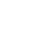# Henry's Law Constants

### Rolf Sander

Atmospheric Chemistry Division

Max-Planck Institute for Chemistry
Mainz, Germany

## Errata

#### Contact, Impressum, Acknowledgements

When referring to the compilation of Henry's Law Constants, please cite this publication:

R. Sander: Compilation of Henry's law constants (version 4.0) for water as solvent, Atmos. Chem. Phys., 15, 4399-4981 (2015), doi:10.5194/acp-15-4399-2015

# Errata

Well, I knew it would happen... A big list like this just couldn't be perfect. I have now added a list of errors that I have made in my compilation.

### Errata for version 4.0

If you notice that any Henry's law data (e.g., from your work) are missing or incorrectly listed, please send an email to rolf.sander@mpic.de.
• When analyzing N2O5 from Fried et al. (1994), I had incorrectly assumed that they were referring to the Henry's law constant $Hscp$ (in M/atm). Re-checking that publication, however, I noticed that they used the dimensionless Henry's law constant $Hscc$. The correct data are: $Hscp$ (N2O5) = 8.7E-4 mol/(m3*Pa) and a temperature-dependence of $d ln{H}_{\mathsf{s}}^{\mathsfit{cp}}/ d \left(1/\mathsfit{T}\right)$ = 3600 K.
• Although I correctly cited the value of $Hscp$(HOBr) > 1.3E2 M/atm from the JPL Data Evaluation (2011), it turned out that the value given there was incorrect. More recent versions of the JPL Data Evaluation present the corrected value of $Hscp$(HOBr) > 1.3E3 M/atm.
• Due to a unit conversion error, all H′ values (products of Henry's law and acidity constants) for strong acids in my compilation were off by a factor of 1000. The corrected data are:  H′(HNO3) = 2.6×107×exp(8700 K ((1)/(T)−(1)/(T⊖))) mol2/(m6 Pa) (Clegg and Brimblecombe, 1990) H′(HNO3) = 2.4×107×exp(8700 K ((1)/(T)−(1)/(T⊖))) mol2/(m6 Pa) (Brimblecombe and Clegg, 1989) H′(HF) = 9.4×101×exp(7400 K ((1)/(T)−(1)/(T⊖))) mol2/(m6 Pa) (Brimblecombe and Clegg, 1989) H′(HCl) = 2.0×107 mol2/(m6 Pa) (Clegg and Brimblecombe, 1986) H′(HCl) = 2.0×107×exp(9000 K ((1)/(T)−(1)/(T⊖))) mol2/(m6 Pa) (Wagman et al., 1982) H′(HCl) = 2.0×107×exp(9000 K ((1)/(T)−(1)/(T⊖))) mol2/(m6 Pa) (Carslaw et al., 1995) H′(HCl) = 2.0×107×exp(9000 K ((1)/(T)−(1)/(T⊖))) mol2/(m6 Pa) (Brimblecombe and Clegg, 1989) H′(HBr) = 7.0×109×exp(10000 K ((1)/(T)−(1)/(T⊖))) mol2/(m6 Pa) (Wagman et al., 1982) H′(HBr) = 7.1×109×exp(6100 K ((1)/(T)−(1)/(T⊖))) mol2/(m6 Pa) (Chameides and Stelson, 1992) H′(HBr) = 8.2×109×exp(10000 K ((1)/(T)−(1)/(T⊖))) mol2/(m6 Pa) (Carslaw et al., 1995) H′(HBr) = 1.3×1010×exp(10000 K ((1)/(T)−(1)/(T⊖))) mol2/(m6 Pa) (Brimblecombe and Clegg, 1989) H′(HI) = 2.1×1010×exp(9800 K ((1)/(T)−(1)/(T⊖))) mol2/(m6 Pa) (Wagman et al., 1982) H′(HI) = 2.5×1010×exp(9800 K ((1)/(T)−(1)/(T⊖))) mol2/(m6 Pa) (Brimblecombe and Clegg, 1989) H′(MSA) = 6.4×1014 mol2/(m6 Pa) (Brimblecombe and Clegg, 1988)
(the error was found after Yuling Chen pointed out an inconsistency in the data for HF; the data were corrected on 2021-02-07)
• In Tab. 5 of Sander (2015), the derivate $d ln\mathsfit{H}/ d \left(1/\mathsfit{T}\right)$ for $CT2$ should be $-2CT3$, not $-2C/T3$.
• The supplement of Sander (2015) contains several Fortran90 files (output/*.f90) with temperature-dependent definitions of Henry's law constants. Here, the expression (1./298.15-1./T) must be replaced by (1./T-1./298.15).

### Errata for version 3.99 (already corrected in subsequent versions)

• Bug fix for data in table 5 by Pfeifer et al. (2001).
• The CASRN for tribromomethanol is [5405-30-1].

### Errata for version 3 (already corrected in subsequent versions)

• In note 20, the solubility for strong acids should be defind as:
([H+] * [A-]) / p(HA)
and NOT
([H+] + [A-]) / p(HA)
Thanks to J. C. Wheeler for pointing this out to me.
• There's a nasty little error in the sign of the temperature dependence in all tables:
The values given in the third column are
+d ln H / d (1/T)
and NOT
- d ln H / d (1/T)
The solubility decreases with increasing temperature, of course. This is shown correctly in equation (5) on page 3.
• Although not mentioned in the table, it should be noted that values for HCHO refer to effective Henry's law constants that take into account increased solubility due to the formation of the diol:
HCHO + H2O = CH2(OH)2
• Data from Allen et al. (1998) are measured, not estimated.
• The reference Gmehling et al. (1981) should be: J. Gmehling and U. Onken and W. Arlt. Vapor-Liquid Equilibrium Data Collection. D. Behrens and R. Eckermann (eds.). Dechema, Frankfurt/Main Vol. 1a (1981)
• page 15: The value of 1.3E-1 M/atm for 2-methylpentane from Ashworth et al. (1988) was quoted incorrectly by Staudinger and Roberts (1996).
• page 36: The values listed for benzo[a]fluoranthene actually refere to benzo[k]fluoranthene.
• page 38: The CASRN for 1-heptanol is [111-70-6], not [110-70-6].
• page 41: The value for 1,2-ethanediol from Bone et al. (1983) should be 4.1E5 M/atm, not 4.0E6 M/atm.
• page 44: The CASRN for methanal (formaldehyde) is [50-00-0], not [50-50-0].
• page 60: For 2,2-dimethylpyridine, the temperature dependence is 7000 K, not 700 K.
• page 61: For 2-butyl nitrate, both the trivial name "isobutyl nitrate" and the CASRN are wrong. The correct CASRN for 2-butyl nitrate is 924-52-7.
• page 61: The species called tert-butyl nitrate should actually be isobutyl nitrate.
• page 75: The value of 2.2 by Hine and Mookerjee (1975) refers to 1,1,1,2-tetrachloroethane, not to 1,1,2,2-tetrachloroethane.
• page 90: The CASRN for 1-butanethiol is [109-79-5], not [109-75-5].
• page 90: The value of 5.8E-1 by Yaws and Yang (1982) refers to diethyl sulfide, not to diethyl disulfide.
• page 92: The species called 3,4'-PCB is actually 3,4-PCB.
• page 93: The value of 1.4E2 by USEPA (1982) refers to 2,4,6-trichlorophenol, not to 2,4,6-PCB.
Thanks to everyone who informed me about any incorrect data!

# * * *

## Convert Henry's Law Constants

Convert: mol/(m3*Pa) (Hscp) M/atm (Hscp) 1/atm (Hsxp) mol/(kg*Pa) (Hsbp) mol/(kg*atm) (Hsbp) (dimensionless aq/g) (Hscc) m3*Pa/mol (Hvpc) m3*atm/mol (Hvpc) atm (Hvpx) (dimensionless g/aq) (Hvcc) (dimensionless aq/g) (alpha)